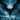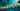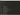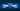Navigate back to the homepage

# 最小化数组分段和问题，算法及其应用(1)LER0ever
October 20th, 2019 · 1 min read1. 从盒子里拿一片加工好的A13 Bionic CPU (为什么是加工好的？因为芯片生产工艺不在中国:)
2. 将A13涂上一些硅脂意思意思
3. 从盒子里拿一片加工好的主板，并将A13摁到主板上
4. 从盒子里拿一个三摄模块，把主板和三摄放到手机后盖上
5. 贴上一块容量非常小并且使用祖传5V2A慢充的电池
6. 用一种非常规的螺丝固定主板和机身，确保你用户拆不开
7. 贴上一块三星产的AMOLED屏幕
8. 屏幕中框强力封胶，确保你用热风枪加热都打不开
9. 试着亮屏，只要没爆炸就算品控过关，放到包装盒里

## 先把终极的问题抛出来:

• 一个人只做一道工序
• 一个人做好几道工序
• 几个人一起做一道工序，这时候需要的时间线性除以人数
• 几个人一起做好几道工序，这时候需要的时间线性除以人数 反正做完之后传给做下一道工序的人就可以了。## 问题 Ver.1### 解法一: 暴力搜索

#### 代码实现

.css-1chxjt6{position:absolute;right:22px;top:24px;padding:8px 12px 7px;border-radius:5px;color:#6f7177;-webkit-transition:background 0.3s ease;transition:background 0.3s ease;}.css-1chxjt6:hover{background:rgba(255,255,255,0.07);}.css-1chxjt6[data-a11y="true"]:focus::after{content:"";position:absolute;left:-2%;top:-2%;width:104%;height:104%;border:2px solid var(--theme-ui-colors-accent,#6166DC);border-radius:5px;background:rgba(255,255,255,0.01);}@media (max-width:45.9375em){.css-1chxjt6{display:none;}}1#include <bits/stdc++.h>2using namespace std;
1int partition(vector<int> A, int n, int k)2{3    if (k == 1)4        return accumulate(A.begin(), A.begin()+n, 0);5    if (n == 1)6        return A;78    int best = INT_MAX;9    for (int j=1; j<=n; j++)10        best = min(best, max(partition(A, j, k-1), accumulate(A.begin()+j, A.begin()+n, 0)));11    return best;12}

### 解法二: 动态规划

#### 代码实现

1int partition(vector<int>& nums, int k) {2    int n = nums.size();3    vector<vector<int>> f(n + 1, vector<int>(k + 1, INT_MAX));4    vector<int> sub(n + 1, 0);5    for (int i = 0; i < n; i++) {6        sub[i + 1] = sub[i] + nums[i];7    }8    dp = 0;9    for (int i = 1; i <= n; i++) {10        for (int j = 1; j <= k; j++) {11            for (int p = 0; p < i; p++) {12                dp[i][j] = min(dp[i][j], max(f[p][j - 1], sub[i] - sub[p]));13            }14        }15    }16    return dp[n][k];17}

### 解法三: 二分查找

#### 代码实现

1int partition(vector<int>& nums, int m) {2    LL l = 0, r = 0;3    int n = nums.size();4    for (int i = 0; i < n; i++) {5        r += nums[i];6        if (l < nums[i]) {7            l = nums[i];8        }9    }10    LL ans =  r;11    while (l <= r) {12        LL mid = (l + r) >> 1;13        LL sum = 0;14        int cnt = 1;15        for (int i = 0; i < n; i++) {16            if (sum + nums[i] > mid) {17                cnt ++;18                sum = nums[i];19            } else {20                sum += nums[i];21            }22        }23        if (cnt <= m) {24            ans = min(ans, mid);25            r = mid - 1;26        } else {27            l = mid + 1;28        }29    }30    return ans;31}

## 问题进化 Ver.3

### More articles from L.E.R Space | Blog### Build jiri for Windows

My experiences and work around for compiling and using jiri under Windows

August 25th, 2018 · 2 min readLink to $https://twitter.com/LER0everLink to$https://github.com/LER0everLink to $https://instagram.com/rongyi.ioLink to$https://www.linkedin.com/in/LER0ever